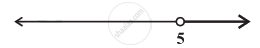# Solution of a linear inequality in variable x is represented on number line given below ______. - Mathematics

MCQ
Fill in the Blanks

Solution of a linear inequality in variable x is represented on number line given below ______.• x ∈ (–∞, 5)

• x ∈ (–∞, 5]

• x ∈ [5, ∞)

• x ∈ (5, ∞)

#### Solution

Solution of a linear inequality in variable x is represented on number line x ∈ (5, ∞).

Explanation:

The gives graph represents all values of x greater than 5 including 5 on the real number line.

So, x ∈ (5, ∞).

Concept: Algebraic Solutions of Linear Inequalities in One Variable and Their Graphical Representation
Is there an error in this question or solution?

#### APPEARS IN

NCERT Mathematics Exemplar Class 11
Chapter 6 Linear Inequalities
Exercise | Q 27 | Page 110
Share